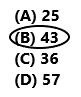# Texas Go Math Grade 5 Lesson 8.4 Answer Key Represent and Solve Multi-Step Problems

Refer to our Texas Go Math Grade 5 Answer Key Pdf to score good marks in the exams. Test yourself by practicing the problems from Texas Go Math Grade 5 Lesson 8.4 Answer Key Represent and Solve Multi-Step Problems.

## Texas Go Math Grade 5 Lesson 8.4 Answer Key Represent and Solve Multi-Step Problems

Unlock the Problem

Shaniqua buys 140 small beads and 30 large beads to make bracelets. She makes 5 bracelets. She uses 13 beads on each bracelet. How many beads does Shaniqua have left?
• Underline the important information.
______________________
140 + 30 = 170
She makes 5 bracelets. She uses 13 beads on each bracelet.
5 x 13 = 65
She used 65 beads total to make 5 bracelets.
170 – 65 = 105
Shaniqua have left with 105 beads.

Example 1

Use multiple single-step equations.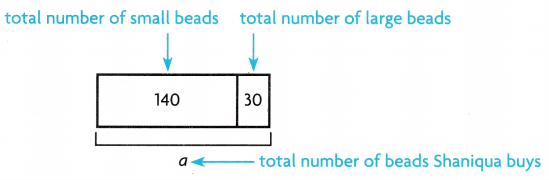140 + 30 = a
___________ = a

STEP 2: Find the total number of beads Shaniqua uses to make 5 bracelets.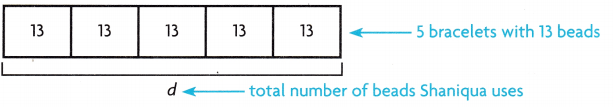5 × 13 = d
___________ = d

STEP 3: Find the total number of beads Shaniqua has left.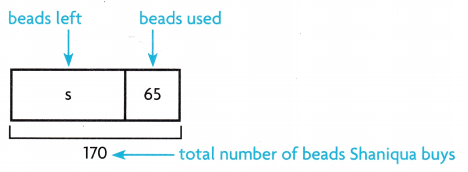170 – 65 = s
_________ = s
So, Shaniqua has _________ beads left.140 + 30 = a
140 + 30 = 170
She makes 5 bracelets. She uses 13 beads on each bracelet.5 × 13 = d
5 x 13 = 65
Here d is the total number of beads Shaniqua uses.
Total number of beads Shaniqua uses to make 5 bracelets is 65 beads.
Total number of beads Shaniqua has left.170 – 65 = s
170 – 65 = 105
So, Shaniqua has 105 beads left.

Try This! Sometimes you can use one multi-step equation to solve a problem.

Miguel sorts his seashell collection into boxes. He has 3 boxes with 15 periwinkle shells in each box. He has 2 boxes with 7 clamshells in each box. He gives his little brother 10 shells. How many shells does he have now?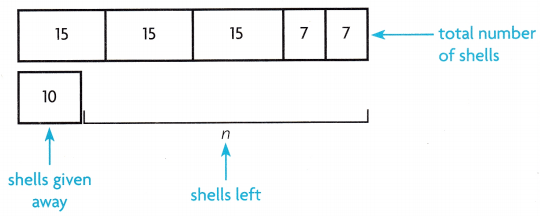3 × 15 + 2 × 7 – 10 = n
________ + ________ – ________= n
________ – ________ = n
________ = n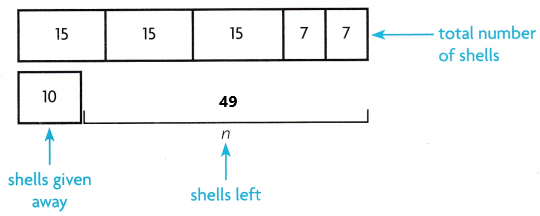3 × 15 + 2 × 7 – 10 = n
45 +14 – 10 = n
59 – 10 = n
49 = n
Explanation:
Miguel sorts his seashell collection into boxes. He has 3 boxes with 15 periwinkle shells in each box. Multiply 3 with 15 the product is 45. He has 2 boxes with 7 clamshells in each box. Multiply 2 with 7 the product is 14. Add 45 with 14 the sum is 59. He gives his little brother 10 shells. Subtract 10 from 59 the difference is 49. Miguel have 49 shells left.

Example 2

Meagan has two card-collection books. The first book has 8 cards on each of 14 pages. The second book has 6 cards on each of 15 pages. Which of the two books has more cards?

STEP 1: Solve the equation b ÷ 8 = 14 to find the number of cards in the first book.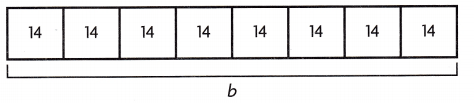Write a related multiplication equation.
14 × _______ = b b = __________

STEP 2: Solve the equation p ÷ 6 = 15 to find the number of cards in the second book.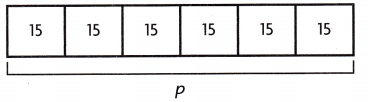Write a related multiplication equation.
15 × _________ = p p = _________

STEP 3: Compare the number of cards in each book.
The first book has _______ cards.
The second book has _______ cards.
Since _______ > _______, the book has more cards.
STEP 1: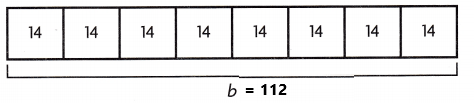The equation b ÷ 8 = 14 is used to find the number of cards in the first book.
The related multiplication equation.
14 × 8 = b
b =112
The number of cards in the first book is 112.

STEP 2:The equation p ÷ 6 = 15 is used to find the number of cards in the second book.
The related multiplication equation.
15 × 6 = p
p = 90
The number of cards in the second book is 90.

STEP 3:
Compare the number of cards in each book.
The first book has 112 cards.
The second book has 90 cards.
Since 112 > 90, the first book has more cards.
Explanation:
Meagan has two card-collection books. The first book has 8 cards on each of 14 pages. The equation b ÷ 8 = 14. The related multiplication is b = 14 x 8. Multiply 14 with 8 the product is 112. The number of cards in the first book is 112. The second book has 6 cards on each of 15 pages. The equation p ÷ 6 = 15. The related multiplication equation is 15 x 6 = p. Multiply 15 with 6 the product is 90. The number of cards in the second book is 90. Since 112 > 90, the first book has more cards.

Math Talk
Mathematical Processes

Explain why you can use a related multiplication to solve a division problem.

Share and Show

Use equations to solve each multi-step problem.

Question 1.
A caterer is making 3 trays of 24 sandwiches and 2 trays of 30 sandwiches. She receives an order for 35 sandwiches. How many sandwiches, s, does the caterer have left?
STEP 1:
A caterer is making 3 trays of 24 sandwiches
3 x 24 = 72
STEP 2:
A caterer is making 2 trays of 30 sandwiches.
2 x 30 = 60
STEP 3:
Total number of sandwiches
72 + 60 = 132
She receives an order for 35 sandwiches.
132 – 35 = s
97 = s
Here s is the sandwiches that the caterer have left.
The caterer have left with 97 sandwiches.
Explanation:
A caterer is making 3 trays of 24 sandwiches. Multiply 3 with 24 the product is 72. A caterer is making 2 trays of 30 sandwiches. Multiply 2 with 30 the product is 60. Add 72 with 60 the sum is 132. Total number of sandwiches are 132. She receives an order for 35 sandwiches. Subtract 35 sandwiches from 132 sandwiches the difference is 97 sandwiches. Here s is the sandwiches that the caterer have left. The caterer have left with 97 sandwiches.

Question 2.
A baseball league has a total of 156 players. The players are divided into 12 equal teams. Each team has 3 coaches. All players and coaches receive 2 jerseys. How many jerseys, j, will each team receive?
STEP 1:
A baseball league has a total of 156 players. The players are divided into 12 equal teams.
156 ÷ 12 = 13
There are 13 players in each team.
STEP 2:

Problem Solving

Use equations to solve.

Question 3.
Multi-Step A florist makes 4 floral arrangements that are exactly the same. He uses 4 bunches of 8 tulips and 2 bunches of 10 daisies. How many flowers, f, are in each arrangement?
He uses 4 bunches of 8 tulips.
4 x 8 = 32
He uses 2 bunches of 10 daisies.
2 x 10 = 20
Total number of flowers in each arrangement is f.
32 + 20 = f
52 = f
In each arrangement there are 52 flowers.
Explanation:
A florist makes 4 floral arrangements that are exactly the same. He uses 4 bunches of 8 tulips and 2 bunches of 10 daisies. Multiply 4 bunches with 8 tulips the product is 32 flowers. Multiply 2 bunches of flowers with 10 daisies the product is 20 flowers. Here total number of flowers in each arrangement is f. Add 32 with 20 the sum is 52. In each arrangement there are 52 flowers.

Question 4.
Multi-Step Francois is rolling coins. He has 3 rolls of 40 quarters. He has 8 rolls of 50 dimes. He exchanges 2 rolls of dimes for 1 roll of quarters. How many coins, c, does Francois have?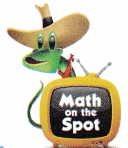Question 5.
Multi-Step Elsa has 4 trays of 15 sedimentary rocks. She has 6 trays of 12 metamorphic rocks. She gives away 5 sedimentary rocks for 7 metamorphic rocks. How many rocks, r, does she have?

Question 6.
Analyze Murial alternates her exercise. She runs 5 miles each day for 3 days and walks 4 miles each day for 2 days in one week. She rides her bike 8 miles each day for 4 days the next week. How many more miles, m, does Murial travel during the second week than the first week?

Question 7.
Apply A volunteer took 3 cups and 7 quarts of a large bowl of soup home from a cooking class.. How much soup did this volunteer take? (Hint: There are 4 cups in a quart.)
(A) 31 cups
(B) 37 cups
(C) 31 quarts
(D) 42 quartsThere are 4 cups in a quart.
7 x 4 cups = 28 cups
There are 28 cups in 7 quarts.
3 cups + 28 cups = 31 cups
Volunteer took 31 cups of soup.
So, option A is correct.
Explanation:
A volunteer took 3 cups and 7 quarts of a large bowl of soup home from a cooking class. There are 4 cups in a quart. Here we have 7 quarts of soup. Multiply 4 cups with 7 the product is 28 cups. Add 28 cups with 3 cups the sum is 31 coups. Volunteer took 31 cups of soup. So, option A is correct.

Question 8.
What is the first step in solving the equation 4 + 10 × 3 – 2 = n?
(A) Find 4 + 10.
(B Find 3 – 2.
(C) Find 10 × 3.
(D) Subtract n.Option c is correct.
Explanation:
The first step in solving the equation 4 + 10 × 3 – 2 = n. The first step is we have to find out 10 x 3. So, option C is correct.

Question 9.
Multi-Step Evelyn has 4 boxes with 8 pieces of sidewalk chalk in each box. She has 3 boxes with 9 stickers in each box. She gets 5 more pieces of sidewalk chalk from her brother. If she places everything into a bin, how many items does she place in the bin?
(A) 24
(B) 55
(C) 64
(D) 72Equation:
4 x 8 + 3 x 9 + 5 = b
32 + 27 + 5 = b
64 = b
Here b is the number of items she places in the bin.
She places 64 items in the bin.
So, option C is correct.
Explanation:
Evelyn has 4 boxes with 8 pieces of sidewalk chalk in each box. She has 3 boxes with 9 stickers in each box. She gets 5 more pieces of sidewalk chalk from her brother. The equation is 4 x 8 + 3 x 9 + 5 = b. The value of b is 64. Here b is the number of items she places in the bin. She places 64 items in the bin. So, option C is correct.

Texas Test Prep

Question 10.
The cost of 3 pizzas is shared equally by 6 people. Each pizza costs $9. Each person contributes$5. Which equation could be used to find, m, the amount of money left?
(A) 3 × 9 – 6 × 5 = m
(B) 9 ÷ 3 – 6 × 5 = m
(C) 6 × 5 – 3 × 9 = m
(D) 3 × 9 + 5 × 6 = m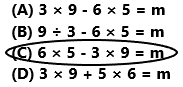Equation:
6 x 5 – 3 x 9 = m
30 – 27 = m
3 = m
Here m is the amount of money left.
The amount of money left is 3.
So, option C is correct.
Explanation:
The cost of 3 pizzas is shared equally by 6 people. Each pizza costs $9. Each person contributes$5. The equation is 6 x 5 – 3 x 9 = m. The value of m is 3. Here m is the amount of money left. The amount of money left is 3. So, option C is correct.

### Texas Go Math Grade 5 Lesson 8.4 Homework and Practice Answer Key

Use equations to solve.

Question 1.
Last week, Mary picked 4 baskets of 8 tomatoes from her garden. This week, she picked 3 baskets of 13 tomatoes. How many more tomatoes, t, does Mars’ pick this week than last week?
Last week, Mary picked 4 baskets of 8 tomatoes from her garden.
4 x 8 = 32
Last week she picked 32 tomatoes from her garden.
This week, she picked 3 baskets of 13 tomatoes.
3 x 13 = 39
This week she picked 39 tomatoes from her garden.
39 – 32 = t
39 – 32 = 7
Mars’ picked 7 more tomatoes this week than last week.
Explanation:
Last week, Mary picked 4 baskets of 8 tomatoes from her garden. Multiply 4 with 8 the product is 32 tomatoes. Last week she picked 32 tomatoes from her garden. This week, she picked 3 baskets of 13 tomatoes. Multiply 3 with 13 the product is 39. This week she picked 39 tomatoes from her garden. Subtract 32 tomatoes from 39 the difference is 7. Mars’ picked 7 more tomatoes this week than last week

Question 2.
A costume designer sews 4 rows of 16 beads on a princess costume, 5 rows of 15 beads on a butterfly costume, and 3 rows of 17 heads on a ballerina costume. How many more heads, b, are sewn on the costume with the most beads than are sewn on the costume with the least number of beads?

Question 3.
Venancio needs to paint 8 game boards for the 4. carnival. Each game board is divided into 12 parts. He paints 5 parts of 8 game boards yellow, 3 parts of 6 game boards red, 2 parts of 7 game boards green, and 15 parts purple. How many more parts, p, does Venancio have left to paint?

Question 4.
Mr. Gates buys frozen yogurt bars for the grade5 picnic. He buys 3 packages of 12 strawberry yogurt bars and 6 packages of 8 banana yogurt bars. He gives an equal number of frozen yogurt bars to each of 3 classes. How many frozen yogurt bars, y, does each class get?

Problem Solving

Question 5.
Rusty has a cap collection. He has 2 shelves with 9 football team caps on each shelf. He has 3 shelves with 11 baseball caps on each shell. He gives his best friend 2 football caps and 3 baseball caps. How many caps, c, does Rusty have now?
He has 2 shelves with 9 football team caps on each shelf.
2 x 9 = 18
Rusty have 18 football team caps.
He has 3 shelves with 11 baseball caps on each shell.
3 x 11 = 33
He have 33 baseball caps.
He gives his best friend 2 football caps and 3 baseball caps.
18 – 2 + 33 – 3 = 46
Now, Rusty have 46 caps.
Explanation:
Rusty has a cap collection. He has 2 shelves with 9 football team caps on each shelf. Multiply 2 with 9 the product is 18. Rusty have 18 football team caps. He has 3 shelves with 11 baseball caps on each shell. Multiply 3 with 11 the product is 33. He have 33 baseball caps. He gives his best friend 2 football caps and 3 baseball caps. Subtract 5 from 51 the difference is 46. Now, Rusty have 46 caps.

Question 6.
Erin pays $3 for each of 4 headbands and$2 for each of 5 barrettes. How much more money, m, does Erin pay for all the headbands than for all the barrettes?
Erin pays $3 for each of 4 headbands.$3 x 4 = $12 Erin pays$2 for each of 5 barrettes.
$2 x 5 =$10
$12 –$10 = $2 Erin pays more$2 for all the headbands than for all the barrettes.
Explanation:
Erin pays $3 for each of 4 headbands and$2 for each of 5 barrettes. First Multiply $3 with 4 the product is$12. Erin Pays $12 for headbands. Next multiply$2 with 5 the product is $10. Erin pays$10 for barrettes. Subtract $10 from$12 the difference is $2. Erin pays more$2 for all the headbands than for all the barrettes.

Lesson Check

Question 7.
Marty is using balloons to decorate for a party. She has 3 groups of 5 pink balloons and 4 groups of 3 yellow balloons. She also has 6 white balloons. Which equation could be used to find, b, the total number of balloons?
(A) 3 × 5 + 4 × 3 = b
(B) 3 + 5 + 4 + 3 + 6 = b
(C) 3 × 5 + 4 × 3 + 6 = b
(D) 3 × 5 × 4 × 3 = b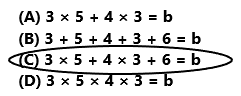Equation
3 x 5 + 4 x 3 + 6 = b
15 + 12 + 6 = b
33 = b
The total number of balloons are 33.
So option C is correct.
Explanation:
Marty is using balloons to decorate for a party. She has 3 groups of 5 pink balloons and 4 groups of 3 yellow balloons. She also has 6 white balloons. The total number of balloons is calculated by this equation. The equation is 3 x 5 + 4 x 3 + 6 = b. So option C is correct.

Question 8.
For the bake sale, 6 people each bake two dozen oatmeal cookies. Each person eats 2 cookies and then delivers the remaining cookies to the bake sale. Which equation could be used to rind, c, the number of oatmeal cookies at the bake sale?
(A) 6 × 2 × 12 – 6 × 2 = c
(B) 6 × 12 – 6 × 2 = c
(C) 2 × 12 – 6 × 2 = c
(D) 6 × 2 – 6 × 2 = c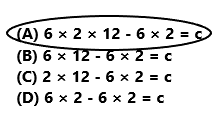Equation
6 x 2 x 12 – 6 x 2 = c
144 – 12 = c
132 = c
The number of oatmeal cookies at the bake sale is 132.
So, option A is correct.
Explanation:
For the bake sale, 6 people each bake two dozen oatmeal cookies. Each person eats 2 cookies and then delivers the remaining cookies to the bake sale. The number of oatmeal cookies at the bake sale is calculated by this equation 6 x 2 x 12 – 6 x 2 = c. So option A is correct.
Question 9.
Jasper used 15 inches of blue ribbon, 14 inches of yellow ribbon, and 3 feet of red ribbon to decorate his box of cookies. How many inches of ribbon did Jasper use?
(A) 65 inches
(B) 32 inches
(C) 93 inches
(D) 29 inches1 feet = 12 inches
3 feet = 36 inches
15 inches + 14 inches + 36 inches = 65 inches
Jasper used 65 inches of ribbon.
So, option A is correct.
Explanation:
Jasper used 15 inches of blue ribbon, 14 inches of yellow ribbon, and 3 feet of red ribbon to decorate his box of cookies. we know that 3 feet is equal to 36 inches. Add 15 inches with 14 inches and 36 inches the sum is 65 inches. Jasper used 65 inches of ribbon. So, option A is correct.

Question 10.
William used 10 ounces of plain milk and 4 pints of chocolate milk when making the restaurant milkshake recipe. How many ounces of milk did William use? (Hint: there are 16 ounces in a pint.)
(A) 64 ounces
(B) 40 ounces
(C) 26 ounces
(D) 74 ounces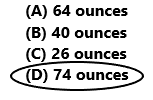Here 1 pint is equal to 16 ounces.
10 ounces + 4 x 16 ounces = m
10 ounces + 64 ounces = m
74 ounces = m
William used 74 ounces of milk.
So, option D is correct.
Explanation:
William used 10 ounces of plain milk and 4 pints of chocolate milk when making the restaurant milkshake recipe. Here 1 pint is equal to 16 ounces. Multiply 4 with 16 ounces the product is 64 ounces. Add 10 ounces with 64 ounces the sum is 74 ounces. William used 74 ounces of milk. So, option D is correct.

Question 11.
Multi-Step Rona has 4 packs of 12 glitter pens. She has 7 packs of 8 scented pens. She gives her friend a pack of glitter pens in exchange for a new pack of scented pens. How many pens does Rona have now?
(A) 92
(B) 104
(C) 100
(D) 112Rona has 4 packs of 12 glitter pens.
4 x 12 = 48
Rona has 48 glitter pens.
She has 7 packs of 8 scented pens.
7 x 8 = 56
She has 56 scented pens.
48 + 56 = 104
She has total 104 pens.
104 – 12 + 8 = 100
Now Rona have 100 pens.
So, option C is correct.
Explanation:
Rona has 4 packs of 12 glitter pens. Multiply 4 with 12 the product is 48. She has 7 packs of 8 scented pens. Multiply 7 with 8 the product is 56. She gives her friend a pack of glitter pens in exchange for a new pack of scented pens. Subtract 12 from 104 the difference is 92. Add 92 pens with 8 pens the sum is 100 pens. Now Rona have 100 pens. So, option C is correct.

Question 12.
Multi-Step Bella has potted plants on her patio. She has 4 pots with 3 begonia plants in each pot. She has 3 pots with 8 petunias in each pot. She plants 7 more zinnia plants in another pot. How many plants does Bella have on her patio?
(A) 25
(B) 43
(C) 36
(D) 57